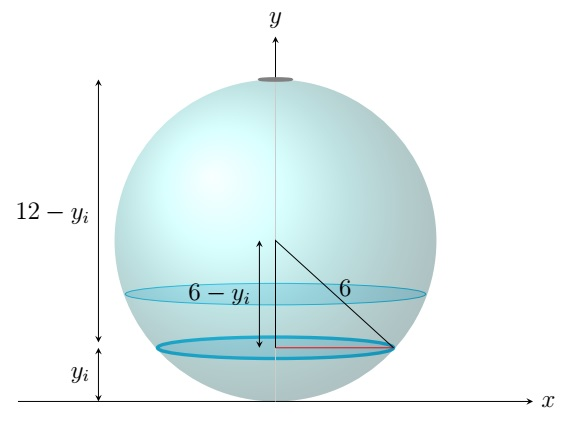Calculus II Home

## Work

Suppose $$f$$ is a continuous function on $$[a,b]$$. Suppose a particle is moving on the $$x$$-axis under a force field that exerts force $$f(x)$$ when the particle is at $$x$$. Then the work done $$W$$ in moving the particle from $$x=a$$ to $$x=b$$ is $W=\int_a^b f(x)\;dx.$

Break $$[a,b]$$ into $$n$$ subintervals $$[x_0,x_1],[x_1,x_2],\ldots,[x_{n-1},x_n]$$ where $$x_i=a+i \Delta x$$ and $$\Delta x=(b-a)/n$$. Choose $$x_i^*$$ from $$[x_{i-1},x_i]$$, $$i=1,2,\ldots,n$$. When the particle is on $$[x_{i-1},x_i]$$, the force acting on the particle is approximately $$f(x_i^*)$$. Then the work done $$W_i$$ in moving the particle from $$x_{i-1}$$ to $$x_i$$ is approximately $$f(x_i^*) \Delta x$$. Therefore the total work done $$W$$ is $W\approx \sum_{i=1}^n f(x_i^*) \Delta x.$ This approximation of $$W$$ gets better as $$n\to \infty$$. Thus $W=\lim_{n\to \infty} \sum_{i=1}^n f(x_i^*) \Delta x =\int_a^b f(x)\;dx.$

Example. A force of $$\sin\left( \frac{\pi x}{4} \right)$$ Newtons is acting on a particle moving on a line when it is $$x$$ meters from the origin. How much work is required in moving the particle from $$x=1$$ to $$x=9$$?

Solution. Here the force is $$f(x)=\sin\left( \frac{\pi x}{4} \right)$$ N when the particle is $$x$$ m from the origin on the $$x$$-axis. Thus required work is $\int_1^9 \sin\left( \frac{\pi x}{4} \right)\;dx =\left.-\frac{4}{\pi}\cos\left( \frac{\pi x}{4} \right) \right\vert_1^9 =-\frac{4}{\pi}\cos\left( \frac{9\pi}{4} \right)+\frac{4}{\pi}\cos\left( \frac{\pi}{4} \right) =-\frac{4}{\pi\sqrt{2}}+\frac{4}{\pi\sqrt{2}}=0.$

Now we discuss the problems of finding the work required to stretch or compress a spring.

Hooke's Law: The force $$f$$ required to maintain a spring stretched or compressed $$x$$ units beyond its natural length is $$f(x)=kx$$ where $$k>0$$ is the spring constant.

Suppose the natural length of a spring is $$x_0$$ m and $$k$$ is the spring constant. Then the work required to stretch it from $$x_1$$ m to $$x_2$$ m is $\int_{x_1-x_0}^{x_2-x_0} f(x)\;dx =\int_{x_1-x_0}^{x_2-x_0} kx\;dx \;J,$ where $$J=Nm$$.

Example. A spring has a natural length of $$20$$ cm and $$15$$ N force is required to keep it stretched to a length of $$25$$ cm. How much work is required to stretch it from $$20$$ cm to $$30$$ cm?

Solution. Since $$15$$ N force is required to stretch $$0.25-0.2=0.05$$ m, by Hooke's Law, $15=f(0.05)=k\cdot 0.05 \implies k=\frac{15}{0.05}=300 \;\text{N/m}.$ Then $$f(x)=300x$$. The work required to stretch the spring from $$0.2$$ m to $$0.3$$ m is $\int_{0.2-0.2}^{0.3-0.2} 300x\;dx=\int_0^{0.1} 300x\;dx=\left. 150x^2 \right\vert_0^{0.1}=1.5 \;J.$

Now we discuss the problems of finding the work required to pump liquid out of a tank.

Example. Consider a spherical tank of radius $$6$$ m. Suppose the tank is filled with a liquid with density $$1200$$ kg/$$m^3$$ to a height of $$4$$ m. Find the work required to empty the tank through a whole at the top of the tank. Assume $$g=9.8$$ m/s$$^2$$.

Solution. First we approximate the required work $$W$$ by approximating the work required to pump a thin layer of liquid to the top of the tank. Draw the origin at the bottom of the sphere and the $$y$$-axis vertically up through the center of the sphere.

Since the tank is filled to a height of $$4$$ m, we partition the liquid into $$n$$ layers by breaking $$[0,4]$$ into $$n$$ subintervals $$[y_0,y_1],[y_1,y_2],\ldots,[y_{n-1},y_n]$$ where $$y_i=i \Delta y$$ and $$\Delta y=4/n$$. The $$i$$th layer of the liquid is approximately a circular cylinder of radius $$\sqrt{6^2-(6-y_i)^2}=\sqrt{12y_i-y_i^2}$$ and height $$\Delta y$$. So the volume $$V_i$$ of the $$i$$th layer is $$V_i\approx \pi (12y_i-y_i^2) \Delta y$$ and its mass $$m_i$$ is $m_i =1200V_i \approx 1200 \pi (12y_i-y_i^2) \Delta y.$The force $$f_i$$ on the $$i$$th layer to overcome the gravitational force on it is $f_i= m_i g \approx 9.8\cdot 1200 \pi (12y_i-y_i^2) \Delta y=11760 \pi (12y_i-y_i^2) \Delta y.$ Then the work $$W_i$$ required in pumping the $$i$$th layer to the top of the tank is $W_i\approx f_i(12-y_i) \approx 11760 \pi (12y_i-y_i^2)(12-y_i) \Delta y=11760 \pi (144y_i-24y_i^2+y_i^3) \Delta y.$ Therefore the total work $$W$$ required is $W= \sum_{i=1}^n W_i \approx \sum_{i=1}^n 11760 \pi (144y_i-24y_i^2+y_i^3) \Delta y.$ This approximation of $$W$$ gets better as $$n\to \infty$$. Thus \begin{align*} W=\lim_{n\to \infty} \sum_{i=1}^n 11760 \pi (144y_i-24y_i^2+y_i^3) \Delta y &=\int_0^4 11760 \pi (144y-24y^2+y^3)\;dy\\ &=11760 \pi\int_0^4 (144y-24y^2+y^3)\;dy\\ &=11760 \pi \left.\left(72y^2-8y^3+\frac{y^4}{4}\right) \right\vert_0^4\\ &=8279040 \pi \;J. \end{align*}

Last edited